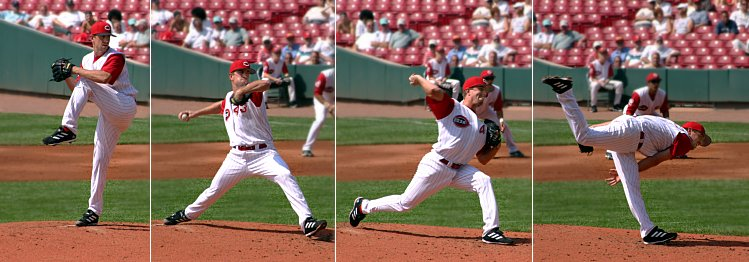# Inertia

Inertia is the resistance of any object due to changes in its motion or direction. There are effectively two types of inertia—mass, which is how much of an object exists (often measured in units like kilograms) and rotational inertia. Objects with high mass have more inertia, which means that more energy is associated with their motion. This takes the form of mass in the kinetic energy expression:

More mass means that it takes more energy to get an object moving (which is part of why an SUV uses more gasoline than a car), and more effort to stop (which is why it takes more braking to stop a car loaded with several people and luggage than one carrying just the driver).

Moment of inertia, also called rotational inertia, , is more complicated since it depends both on mass and shape. Rotational inertia (called the moment of inertia by physicists) is an important part of rotational kinetic energy. The equation for rotational kinetic energy is quite similar:

is how fast the object spins around.Figure 1. Throwing the baseball means the pitcher exerts a force on the ball. The inertia of the ball resists the change in motion. Spinning the ball does the same, but it's the rotational inertia that resists motion (the torque in this case).